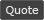WoWInterface - View Single Post - Help with if and 'and not'
 Thread: Help with if and 'and not' View Single Post
 10-27-16, 06:39 PM #4 MunkDev A Scalebane Royal Guard Join Date: Mar 2015 Posts: 427 Lua Code: `firstArgument = truesecondArgument = false if not (firstArgument and secondArgument) then print(1)    -- This is true, because the arguments in the    -- parenthesis are resolved first.     -- Since secondArgument is false, the overall outcome inside    -- the parenthesis will also be false.    -- Once it gets back outside the parenthesis, the statement is negated,    -- meaning it results in the reverse value of the parenthesis outcome.end if not firstArgument and secondArgument then print(2)    -- This is false, because the keyword 'not' will in this case    -- negate the firstArgument, but has no effect on the secondArgument.end if firstArgument and not secondArgument then print(3)    -- This is true, because the secondArgument will be negated into true,    -- and since firstArgument is also true, the overall outcome is true.end if (not firstArgument and secondArgument) then print(4)    -- This is false, because it's not any different from the second    -- example. Since nothing affects the if-case outside the    -- parenthesis, it'll result in the same outcome.end if not (not firstArgument and secondArgument) then print(5)    -- This is true, because the parenthesis returns false, which is then    -- negated into true. firstArgument is negated into false, consolidated    -- with secondArgument into false again, and then negated into true.end if firstArgument or secondArgument then print(6)    -- This is true, because the firstArgument is true.    -- If either argument is true when using 'or', it'll result in true,    -- even if one or more arguments are false.end if secondArgument or firstArgument then print(7)    -- This is true all the same, because even though secondArgument is    -- checked first, firstArgument is still true and thus the outcome is true.end if secondArgument or not firstArgument then print(8)    -- This is false, because both arguments will be false.    -- secondArgument is already false, and firstArgument is negated into false,    -- as explained in the second example.end if ( firstArgument and not secondArgument) or (not firstArgument and secondArgument) then print(9)    -- The first parenthesis results in true, the second parenthesis results in false.    -- Since either argument will do, because we're using 'or', the result is true.end`